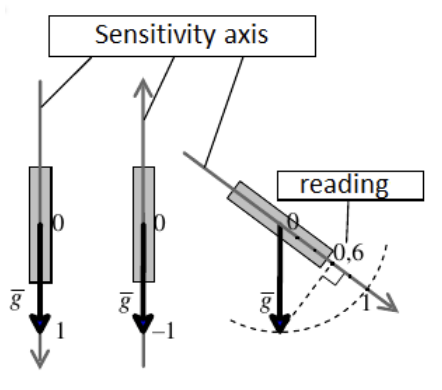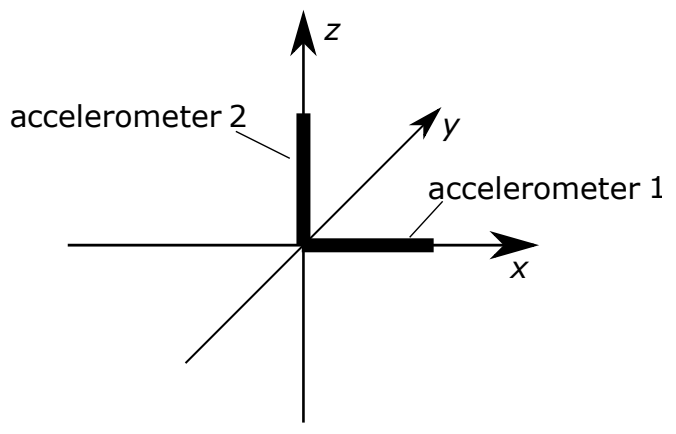시간 제한메모리 제한제출정답맞힌 사람정답 비율
1 초 (추가 시간 없음) 256 MB111100.000%

## 문제

Modern smart devices can serve as GPS navigators and step counters; they can tell whether the user is walking, running, taking a taxi or a bus; they can automatically re-orient the image on the screen, etc. To do all of these and many other things, they rely on the so called accelerometers. A simplest single-axis accelerometer is connected with a direction, which is called its sensitivity axis; readings from a stationary acccelerometer allow to compute the deviation of its axis from a downward vertical, i.e. from the vector of gravity. If there are several single-axis accelerometers mounted on the device, the locations of their axes can be used to define the spatial orientation of the device as a whole.

A perfect accelerometer measures the cosine of the angle between its sensitivity axis and the direction of gravity. If the sensitivity axis is pointed downwards, i.e. along the gravity vector $\overline{g}$, the accelerometer shows the value $1$, if the sensitivity axis is pointed upwards, it shows the value $-1$. If the axis is tilted at an angle to the vertical, the readings of the perfect accelerometer equals the projection of a single gravity vector $\overline{g}$ on the axis:However, the production of the miniature accelerometers is not entirely fault-free. Defects of sensors lead to the following errors in readings:

1. deviation of the sensitivity axis of the accelerometer from the correct direction (bad mounting);
2. proportional change in readings, i.e. increase or decrease in all readings according to a common factor;
3. systemic shift of all readings by some constant;

If there are several accelerometers installed in the device, the errors described above can manifest differently in each of the accelerometers. However, if we examine a series of readings of an accelerometer in different positions, it turns out that the errors affect all of its readings identically.

To figure out the precise nature of the errors in the accelerometer readings in order to correct them digitally, accelerometers are calibrated. One of the ways to calibrate is to collect their readings in several strictly defined positions of the device and to calculate the parameters of the defects described above for each of the accelerometers of the device. These parameters can be then applied to compute the orientation of the device in arbitrary position.

In this problem, we will examine a device with two rigidly mounted accelerometers. When the device is oriented in space in the standard manner, one of the accelerometers must point to the right along the axis $X$, and the other one must point up along the axis $Z$ (opposite to gravity). The user can rotate the device: assume that rotation is only possible in the plane $XZ$. In this case, the orientation of the device is defined completely by the angle of counter-clockwise rotation around the axis $Y$ from the standard orientation (counter-clockwise means from the axis $X$ to the axis $Z$).To calibrate such an accelerometer, the accelerometer readings are taken when the device is rotated by degrees divisible by 90, which allows to define all parameters of the defects.

Based on the provided calibration datasets, find out the parameters of the defects and find a way to produce the real position of the device based on the accelerometer readings.

## 입력

The first four lines of the input file contain the readings of accelerometers when device is rotated by $0$, $90$, $180$ and $270$ degrees, respectively.

The next line of the input file contains an integer $T$ --- the number of readings, for which the position of the device must be found ($1$ $\le$ $T$ $\le$ $1\,000$). Each of the remaining $T$ lines contains accelerometer readings for the device orientation which must be computed.

Each reading contains two space-separated real numbers --- the readings of the first accelerometer (oriented along the axis $X$) and of the second accelerometer (oriented along the axis $Z$), respectively. Both numbers are given with 15 digits after the decimal point.

It is guaranteed that the readings in the input file have been acquired using the model of measurements and errors described in the problem statement. Errors in readings cannot be too big: the deviation of the sensitivity axis from the correct direction is never greater than $30^{\circ}$, and the proportional change of readings never changes them more than two-fold, and the shift is never greater than $5$ in absolute value.

## 출력

In the output file, print $T$ numbers, one per line. Each number is the turning angle for the device in degrees for the corresponding accelerometers readings. The turning angle must be within the range of $0^{\circ}$ to $360^{\circ}$ inclusive. The relative or absolute error of each answer must not exceed $10^{-6}$.

## 예제 입력 1

0 -1
-1 0
0 1
1 0
5
-0.707106781186547 -0.707106781186547
-0.707106781186547 0.707106781186547
0.707106781186547 0.707106781186547
0.707106781186547 -0.707106781186547
-0.866025403784438 -0.5


## 예제 출력 1

45
135.00
225
315
60


## 예제 입력 2

-0.091012995433623 -0.946575228282571
-0.983288528313429 0.028440168472892
0.291012995433623 0.846575228282571
1.183288528313429 -0.128440168472892
5
-0.801067248717891 -0.628508848717885
-0.530934079986151 0.639439998807080
1.001067248717891 0.528508848717886
0.730934079986151 -0.739439998807080
-0.933661882864499 -0.430356435566630


## 예제 출력 2

45.000000000000043
135.000000000000000
225.000000000000000
315.000000000000000
59.999999999999986


## 노트

In the first example, there are no defects in the accelerometers.

In the second example, all three error components influence each of the two accelerometers, and to a different degree.